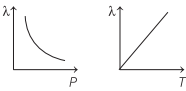# Mean Free Path Physics | Definition, Formula – Kinetic Theory of Gases

Mean Free Path Definition Physics:
The average distance travelled by a molecule between two successive collisions is called mean free path (λ).

We are giving a detailed and clear sheet on all Physics Notes that are very useful to understand the Basic Physics Concepts.

## Mean Free Path Physics | Definition, Formula – Kinetic Theory of Gases

Mean Free Path Formula Physics:
Mean free path is given by

λ = $$\frac{k T}{\sqrt{2} \pi \sigma^{2} p}$$where,
σ = diameter of the molecule,
p = pressure of the gas,
T = temperature and
k = Boltzmann’s constant.

Mean free path, λ ∝ T and

Mean Free Path is Inversely Proportional to,

λ ∝ $$\frac{1}{p}$$

### Mean Free Path in Kinetic Theory of Gases

On the basis of kinetic theory of gases, it is assumed that the molecules of a gas are continuously colliding against each other. Mean Free Path is the average distance traversed by molecule between two successive collisions.

Kinetic Theory of Gases:
In this concept, it is assumed that the molecules of gas are very minute with respect to their distances from each other. The molecules in gases are in constant, random motion and frequently collide with each other and with the walls of any container.

In this portion, you will learn about the properties of gases, based on density, pressure, temperature and energy. Continue reading here to learn more.